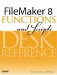# Atan()

Category: Trigonometric

 Syntax: Atan ( number )

Parameters:

numberAny expression that resolves to a numeric value.

Data type returned: Number

Description:

The arc tangent of a number is the angle (measured in radians) whose tangent is the specified number. The range of values returned by the Atan function is -(pi/2) to pi/2.

If Atan (x) = y, then Tan (y) = x.

```Atan (x) = Atan (-x).
```

Examples:

```Atan (0) = 0
Atan (1) = .785398163
```

which is pi/4 radians, or 45 degrees.FileMaker 8 Functions and Scripts Desk Reference
ISBN: 0789735113
EAN: 2147483647
Year: 2004
Pages: 352